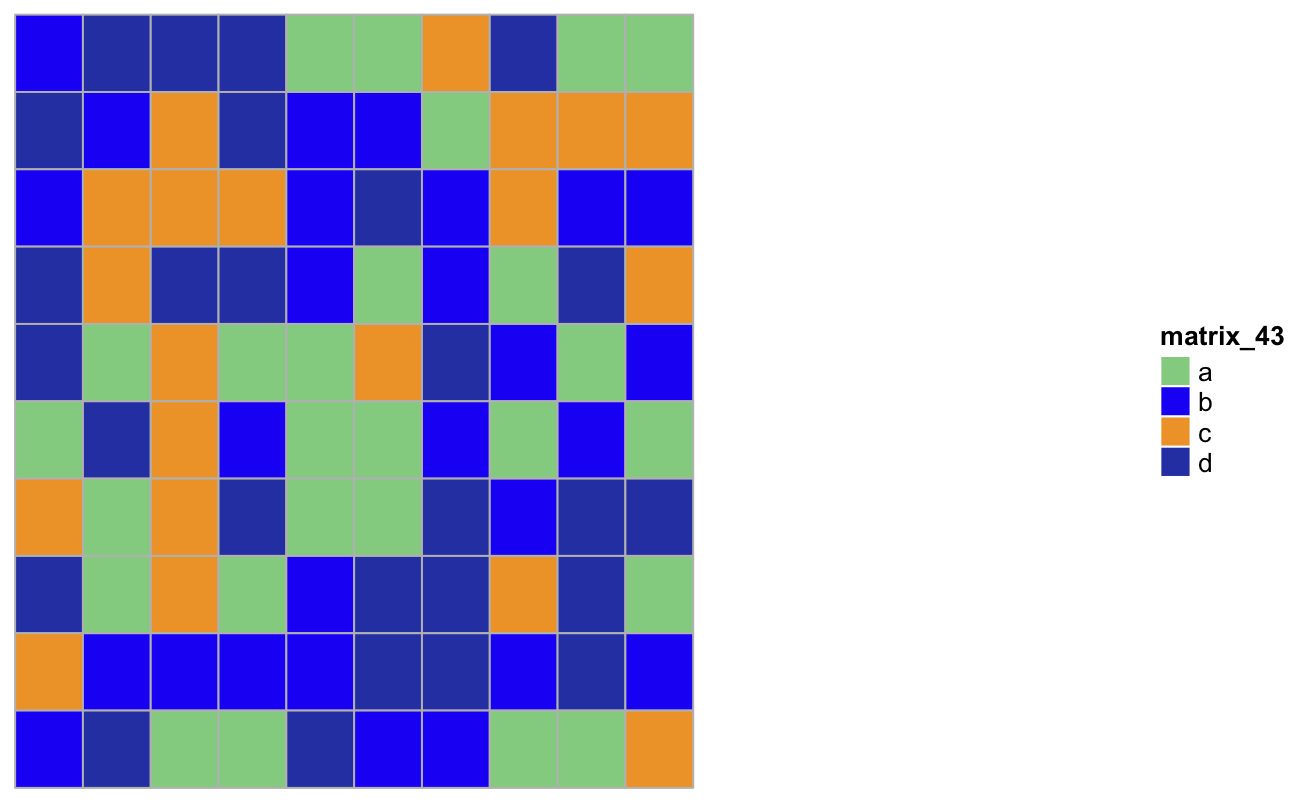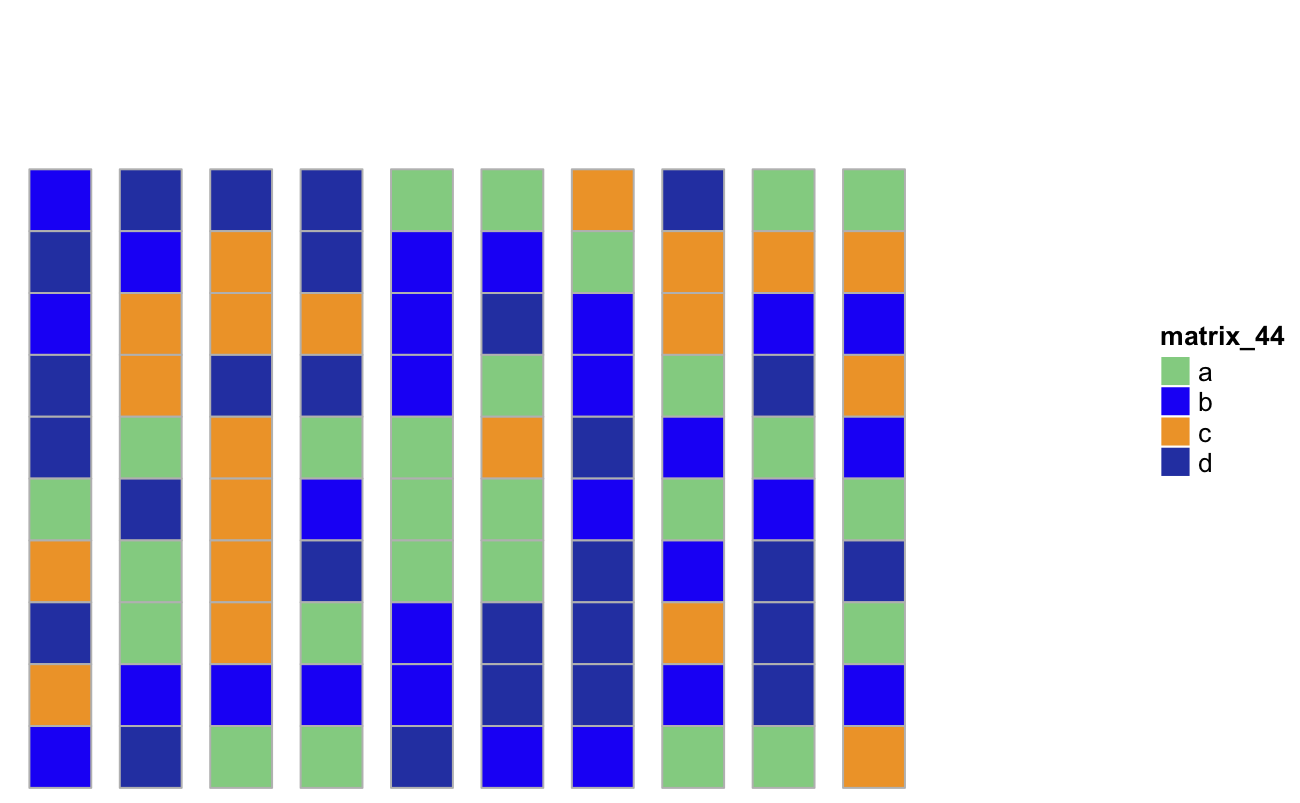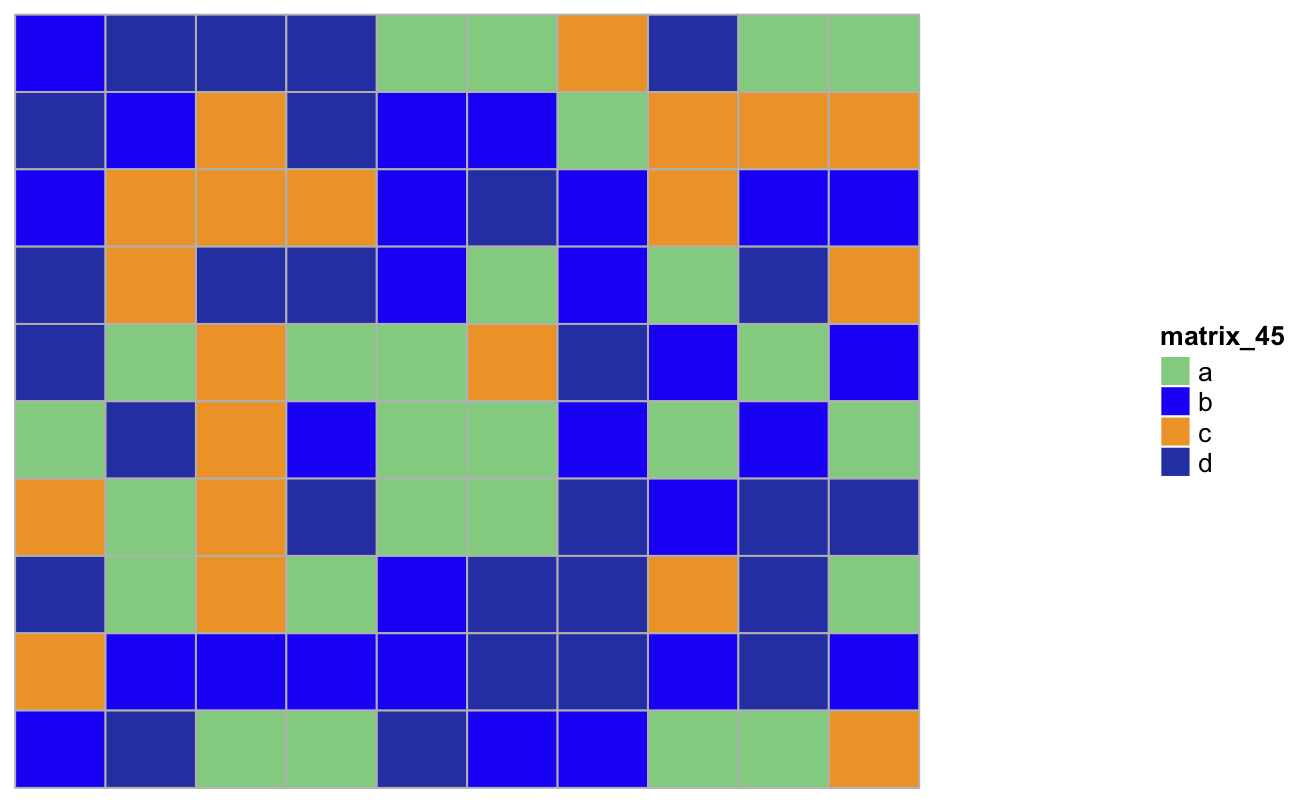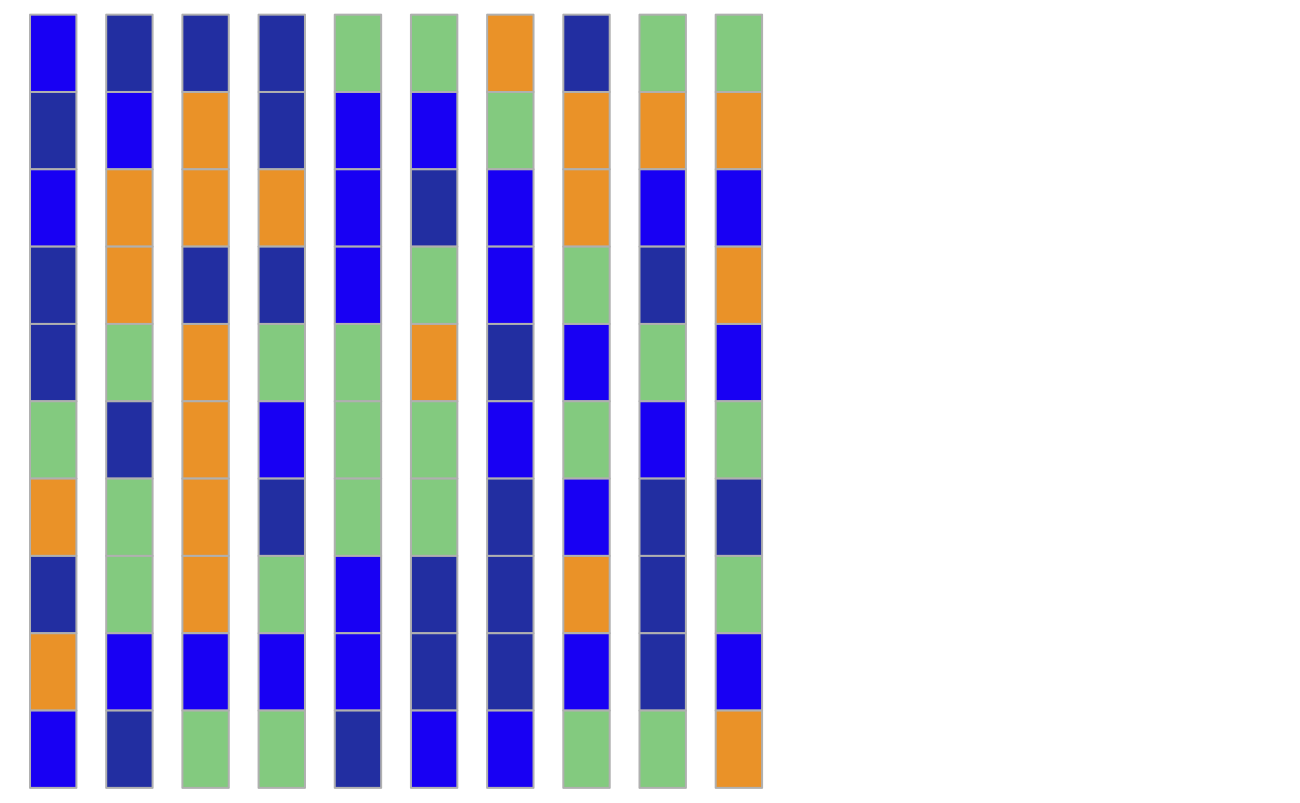## Monday, January 30, 2017

### How to make a heatmap using character matrix

People are willing to read colors. I will show you how to repesent characters in heatmap. `ComplexHeatmap` can directly translate a character matrix into heatmap. It is good to learn basics of `grid` package which `ComplexHeatmap` and `ggplot2` build on.
`grid.rect` is the function used to draw rectangles.
``````library(ComplexHeatmap)
colors<- structure(circlize::rand_color(4), names = c("a", "b", "c", "d"))
set.seed(123)
mat_letters<- matrix(sample(letters[1:4], 100, replace = TRUE), 10, 10)
## default
Heatmap(mat_letters, col = colors)``````
I want to have some gap between the cells:
``````cell_fun<-  function(j, i, x, y, width, height, fill) {
grid.rect(x = x *0.6, y = y, width = width * 0.6, height = height,
gp = gpar(col = "grey", fill = fill))
}
Heatmap(mat_letters, rect_gp = gpar(type = "none"), cell_fun = cell_fun, col = colors)``````It may look different in an interactive graphical device. save the figure in a pdf and you will see the effect.
``````## always square and have gaps between cells
cell_fun2<- function(j, i, x, y, width, height, fill) {
s = min(unit.c(convertWidth(width, "cm"), convertHeight(height, "cm")))
grid.rect(x = x*0.8, y = y*0.8, width = s * 0.8, height = s*0.8,
gp = gpar(col = "grey", fill = fill))
}
Heatmap(mat_letters, rect_gp = gpar(type = "none"), cell_fun = cell_fun2, col = colors)````````````cell_fun3<-  function(j, i, x, y, width, height, fill) {
grid.rect(x = x*0.8, y = y, width = width * 0.8, height = height,
gp = gpar(col = "grey", fill = fill))
}
Heatmap(mat_letters, rect_gp = gpar(type = "none"), cell_fun = cell_fun3, col = colors)``````## make cells thiner

``````cell_fun4<- function(j, i, x, y, width, height, fill) {
s = min(unit.c(convertWidth(width, "cm"), convertHeight(height, "cm")))
grid.rect(x = x*0.6 , y = y, width = s* 0.6, height = height,
gp = gpar(col = "grey", fill = fill))
}
Heatmap(mat_letters, rect_gp = gpar(type = "none"), cell_fun = cell_fun4, col = colors, show_heatmap_legend = FALSE)``````